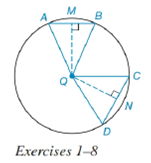Chapter 6.4, Problem 5EElementary Geometry For College St...

7th Edition
Alexander + 2 others
ISBN: 9781337614085

Solutions

Chapter
SectionElementary Geometry For College St...

7th Edition
Alexander + 2 others
ISBN: 9781337614085
Textbook Problem

In Exercises 1 to 8, use the figure provided.If m ∠ C Q D < m ∠ A Q B , write an inequality that compares C D to A B .To determine

To write:

An inequality that compares CD to AB.

Explanation

Given:

mCQD<mAQB and the figure given below.

Theorem:

In a circle (or in congruent circles) containing two unequal central angles, the larger angle corresponds to the larger intercepted arc.

In a circle (or in congruent circles) containing two unequal minor arcs, the greater minor arc corresponds to the longer of the chords related to these arcs.

Calculation:

Using the theorem.

Since the angles are unequal i.e. mCQD<mAQB and from the given figure.

The arc corresponding to angle mCQD is mCD and the arc corresponding to angle mAQB is mAB, we conclude that mCD<mAB

Still sussing out bartleby?

Check out a sample textbook solution.

See a sample solution

The Solution to Your Study Problems

Bartleby provides explanations to thousands of textbook problems written by our experts, many with advanced degrees!

Get Started

4546 Find the limit. limt0t3tan32t

Calculus (MindTap Course List)

Find the mean for the following set of scores: 2, 7, 9, 4, 5, 3, 0, 6

Essentials of Statistics for The Behavioral Sciences (MindTap Course List)

Fill in each blank: 6gal3qt=pat

Elementary Technical Mathematics

Find the area of the region bounded by the given curves. y = x2, y = 4x x2

Single Variable Calculus: Early Transcendentals, Volume I

The polar form for the graph at the right is:

Study Guide for Stewart's Multivariable Calculus, 8th

True or False: If f′(c) = 0 and f″(c) < 0, then c is a local minimum.

Study Guide for Stewart's Single Variable Calculus: Early Transcendentals, 8th